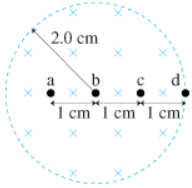# Problem: The magnetic field in the figure is decreasing at the rate of 0.7 T/s.(a) What is the magnitude of the acceleration of a proton at rest at point d? Express your answer as an integer and include the appropriate units.(b) What is the direction of the acceleration of a proton at rest at point d?

###### FREE Expert Solution

$\overline{){\mathbf{a}}{\mathbf{=}}\frac{\mathbf{q}\mathbf{r}}{\mathbf{2}\mathbf{m}}{\mathbf{|}}\frac{\mathbf{d}\mathbf{B}}{\mathbf{d}\mathbf{t}}{\mathbf{|}}}$

Here, q = 1.6 × 10-19 C, m = 1.67 × 10-27 kg, $\mathbf{|}\frac{\mathbf{d}\mathbf{B}}{\mathbf{d}\mathbf{t}}\mathbf{|}$ = 0.7 T/s, and r =  = 2 × 10-2 m.

96% (88 ratings)###### Problem Details

The magnetic field in the figure is decreasing at the rate of 0.7 T/s.(a) What is the magnitude of the acceleration of a proton at rest at point d? Express your answer as an integer and include the appropriate units.

(b) What is the direction of the acceleration of a proton at rest at point d?